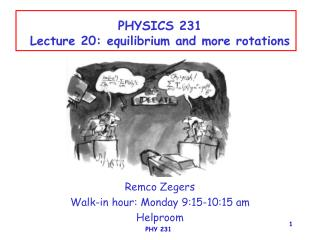# PHYSICS 231 Lecture 20: equilibrium and more rotations - PowerPoint PPT PresentationDownload PresentationPHYSICS 231 Lecture 20: equilibrium and more rotations

PHYSICS 231 Lecture 20: equilibrium and more rotationsDownload Presentation## PHYSICS 231 Lecture 20: equilibrium and more rotations

- - - - - - - - - - - - - - - - - - - - - - - - - - - E N D - - - - - - - - - - - - - - - - - - - - - - - - - - -
##### Presentation Transcript

1. PHYSICS 231Lecture 20: equilibrium and more rotations Remco Zegers Walk-in hour: Monday 9:15-10:15 am Helproom PHY 231

2. gravitation Only if an object is near the surface of earth one can use: Fgravity=mg with g=9.81 m/s2 In all other cases: Fgravity= GMobjectMplanet/r2 with G=6.67E-11 Nm2/kg2 This will lead to F=mg but g not equal to 9.8 m/s2 (see Previous lecture!) If an object is orbiting the planet: Fgravity=mac=mv2/r=m2r with v: linear velocity =angular vel. So: GMobjectMplanet/r2 = mv2/r=m2r Kepler’s 3rd law: T2=Ksr3 Ks=2.97E-19 s2/m3 r: radius of planet T: period(time to make one rotation) of planet Our solar system! PHY 231

3. A child of 40 kg is sitting in a Ferris wheel, rotating with an angular velocity of 0.4 rad/s. The radius of the wheel is 9 m. What is the force exerted by the seat on the child at the top and at the bottom. top: Fcenter=0 -mg+n+mac=0 n=mg-m2r=392-58=334 bottom: Fcenter=0 -mg+n-mac=0 n=mg+m2r=392+58=450 The seat is fixed to the wheel. Its centripetal acceleration is directed towards the center. Top: the seat is accelerated away from the child Bottom: the seat is accelerated towards the child PHY 231

4. Previously Demo: Leaning tower Torque: =Fd Center of Gravity: Translational equilibrium: F=ma=0 The center of gravity does not move! Rotational equilibrium: =0 The object does not rotate Mechanical equilibrium: F=ma=0 & =0 No movement! PHY 231

5. examples: A lot more in the book! Where is the center of gravity? PHY 231

6. Torque: =Fd quiz (extra credit) 40N 20N 40N -2 0 1 2 m • A wooden bar is initially balanced. Suddenly, 3 forces are • applied, as shown in the figure. Assuming that the bar can • only rotate, what will happen (what is the sum of torques)? • the bar will remain in balance • the bar will rotate counterclockwise • the bar will rotate clockwise PHY 231

7. T1 T2 Translational equilibrium F=ma=0 T1+T2-w=0 so T1=w-T2 Rotational equilibrium =0 T10-0.5*w+0.75*T2=0 T2=0.5/0.75*w=2/3w w T1=1/3w T2=2/3w 0 Weight of board: w What is the tension in each of the wires (in terms of w)? PHY 231

8. s=0.5 coef of friction between the wall and the 4.0 meter bar (weight w). What is the minimum x where you can hang a weight w for which the bar does not slide? Translational equilibrium (Hor.) Fx=ma=0 n-Tx=n-Tcos37o=0 so n=Tcos37o Translational equilibruim (vert.) Fy=ma=0 sn-w-w+Ty=0 sn-2w+Tsin37o=0 sTcos370-2w+Tsin370=0 1.00T=2w Rotational equilibrium: =0 xw+2w-4Tsin370=0 so w(x+2-4.8)=0 x=2.8 m sn T Ty n Tx w w (x=0,y=0) PHY 231

9. Demo: fighting sticks PHY 231

10. r Ft=mat Torque and angular acceleration F Newton 2nd law: F=ma m Ftr=mrat Ftr=mr2 Used at=r =mr2 Used =Ftr The angular acceleration goes linear with the torque. Mr2=moment of inertia PHY 231

11. Two masses F =mr2 =(m1r12+m2r22) If m1=m2 and r1=r2 =2mr2 r r m m Compared to the case with only one mass, the angular acceleration will be twice smaller when applying the same torque, if the mass increases by a factor of two. The moment of inertia has increased by a factor of 2. PHY 231

12. Two masses at different radii F =mr2 =(m1r12+m2r22) If m1=m2 and r2=2r1 =5mr2 m r r m When increasing the distance between a mass and the rotation axis, the moment of inertia increases quadraticly. So, for the same torque, you will get a much smaller angular acceleration. PHY 231

13. A homogeneous stick F =mr2 =(m1r12+m2r22+…+mnrn2) =(miri2) =I Moment of inertia I: I=(miri2) m m m m m m m m m m Rotation point PHY 231

14. Two inhomogeneous sticks F F m 5m r m m =(miri2) 118mr2 =(miri2) 310mr2 m m m m 5m m 5m m m m m m m m m 5m 18 m 18m Easy to rotate! Difficult to rotate PHY 231

15. More general. compare with: F=ma The moment of inertia in rotations is similar to the mass in Newton’s 2nd law. =I Moment of inertia I: I=(miri2) PHY 231

16. =I Moment of inertia I: I=(miri2) Answer: A A simple example F F r r A and B have the same total mass. If the same torque is applied, which one accelerates faster? PHY 231

17. The rotation axis matters! I=(miri2) =0.2*0.+0.3*0.52+ 0.2*0+0.3*0.52 =0.3 kgm2 I=(miri2) =0.2*0.52+0.3*0.52+ 0.2*0.52+0.3*0.52 =0.5 kgm2 PHY 231